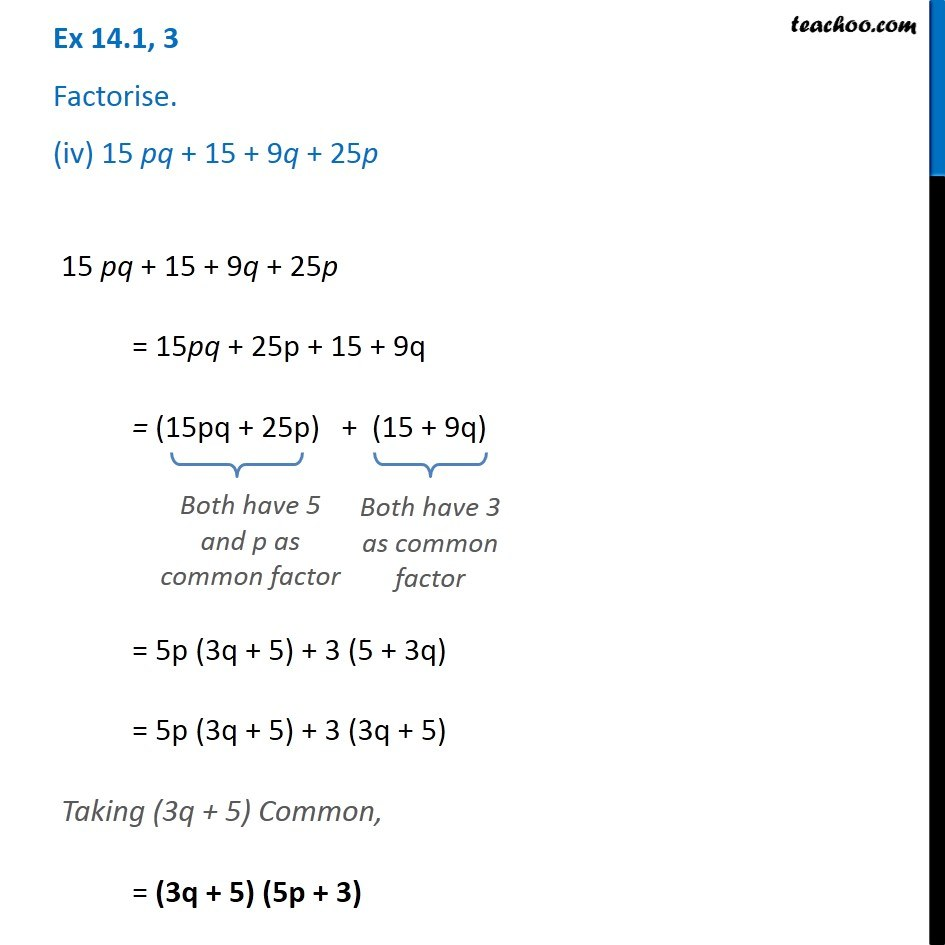1. Chapter 14 Class 8 Factorisation
2. Serial order wise
3. Ex 14.1

Transcript

Ex 14.1, 3 Factorise. (iv) 15 pq + 15 + 9q + 25p 15 pq + 15 + 9q + 25p = 15pq + 25p + 15 + 9q = (15pq + 25p) + (15 + 9q) Both have 5 and p as common factor Both have 3 as common factor = 5p (3q + 5) + 3 (5 + 3q) = 5p (3q + 5) + 3 (3q + 5) Taking (3q + 5) Common, = (3q + 5) (5p + 3)

Ex 14.1